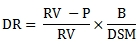# SQL Server pricing function for discount securities

DIS

Updated: 10 October 2014

Use DIS to calculate the price or discount rate for a discount security. The formula for price is:Where:

 B = Number of days in the year DR = Discount Rate DSM = Number of days from settlement date to maturity date P = Price per 100 par value RV = Redemption Value

The formula for discount rate is:Where:

 B = Number of days in the year DR = Discount Rate DSM = Number of days from settlement date to maturity date P = Price per 100 par value RV = Redemption Value

The DIS function allows you to pass values for B/DSM, RV and either DR or P and returns the other.
Syntax
SELECT [wct].[DIS](
<@DSM, float,>
,<@RV, float,>
,<@P, float,>
,<@D, float,>)
Arguments
@DSM
the time in years from settlement to maturity. @DSM is an expression of type float or of a type that can be implicitly converted to float.
@RV
the redemption value. @RV is an expression of type float or of a type that can be implicitly converted to float.
@P
the price of the security. @P is an expression of type float or of a type that can be implicitly converted to float.
@D
the discount rate on the security. @D is an expression of type float or of a type that can be implicitly converted to float.
Return Type
float
Remarks
·         If @DSM is NULL then @DSM = 0.
·         If @RV is NULL then @RV = 100.
·         If @D is NULL and @P is NULL then NULL is returned.
·         If @D is not NULL then the function calculates the price from the inputs otherwise the function calculates the discount rate.
Examples
In this example we calculate the discount rate for a security with a price of 99.72 and redemption value of 100. There are 69 days from settlement to maturity and 365 days in the year.
SELECT
wct.DIS(
69/365E+00 --@DSM
,100.00     --@RV
,99.72      --@P
,NULL       --@D
) as [Discount Rate]
This produce the following result.
Discount Rate
----------------------
0.0148115942028987

In this example we calculate the price for a security with a discount rate of 1.9%. There are 131 days from settlement to maturity and 360 days in the year.
SELECT
wct.DIS(
131/360E+00--@DSM
,10000.00   --@RV
, NULL      --@P
,0.019      --@D
) as [Price]
This produces the following result.
Price
----------------------
9930.86111111111

In this example we calculate the price for a security with a discount rate of 5.5%. There are 190 days from settlement to maturity and 364 days in the year.
SELECT
wct.DIS(
190/364E+00--@DSM
,1000000.00 --@RV
, NULL      --@P
,0.055      --@D
) as [Price]
This produces the following result.
Price
----------------------
971291.208791209

### SupportCopyright 2008-2023 Westclintech LLC         Privacy Policy        Terms of Service